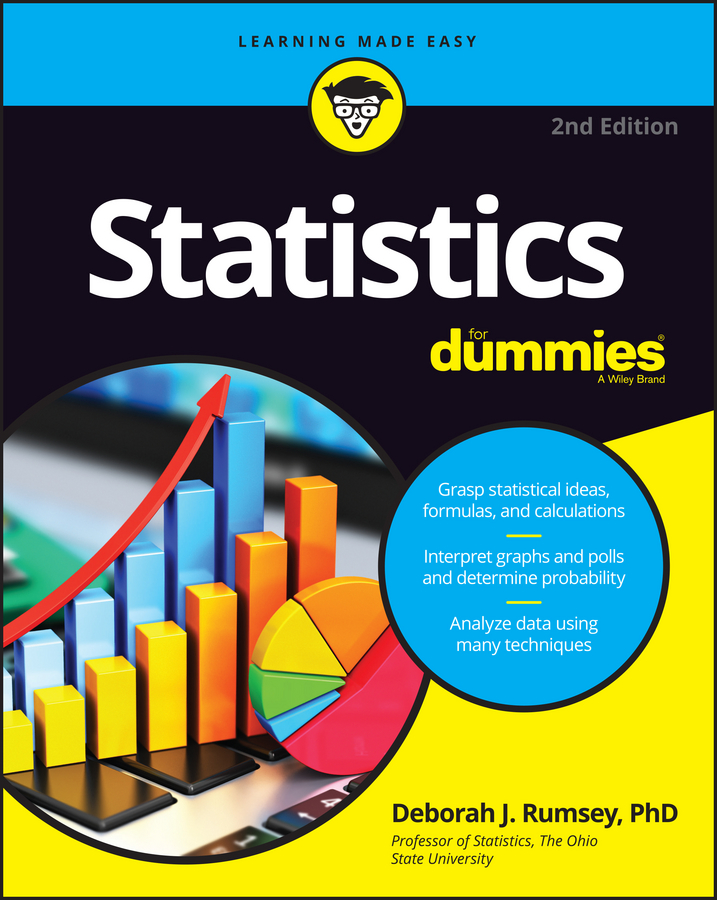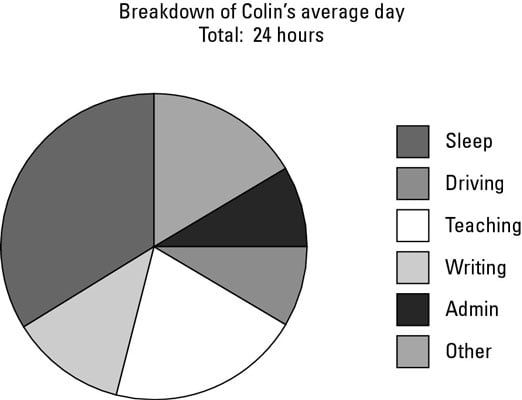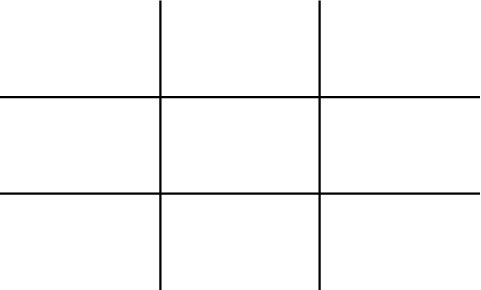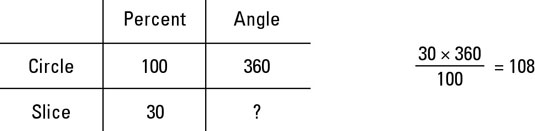##### Statistics For DummiesA pie chart looks – you’ve guessed it – a bit like a pie. The chart is a circle with various-sized slices ‘cut out’ from the middle to the edge. The size of the slices shows the relative size of the categories. You often see a pie chart showing the results of an opinion poll.

Pie charts use angles to show the relative sizes of various categories. Pie charts are circular and are cut into ‘slices’: the bigger the slice of pie, the bigger the group it represents.## Deciding when to use a pie chart

You use a pie chart rather than a bar chart when you’re not very interested in the actual numbers you want to represent but want to see how big the groups are compared with each other.

A good example is if you want to give a presentation about the age groups of your company’s customers but don’t want the audience to know precisely how many customers you have.

You frequently see pie charts on election-night reports on TV to show the distribution of votes. In a very close election race, the slices representing the two front-runners are almost the same size.

## Handling angles, percentages and numbers

You probably won’t be surprised to find that you can work out the values associated with pie charts using the Table of Joy. A whole circle contains 360 degrees. In a pie chart, those 360 degrees correspond to the total of the values represented in the chart.

The Table of Joy is a technique for figuring out what sum you need to do when you have two amounts you know to be proportional – that is, if you double the size of one, you double the size of the other. The Table of Joy looks like an oversized noughts and crosses grid.

When you work with a pie chart, you may need to figure out one of the following three things:
• The size of the angle in a slice.

• The value of a slice.

• The total of the values in all the slices.

To find one of these things, you need to know the other two. Here’s how to use the Table of Joy to work with a pie chart:
1. Draw out a noughts-and-crosses grid.Leave yourself plenty of room in the grid for labels.

2. Label the top row with ‘value’ and ‘degrees’.

Label the sides with ‘slice’ and ‘circle’.

3. Write 360 in the ‘circle/degrees’ cell and the two other pieces of information you have in the appropriate places.

Put a question mark in the remaining cell.

4. Write down the Table of Joy sum.

The sum is the number in the same row as the question mark times by the number in the same column, all divided by the number opposite.

5. Work out the sum.

The answer is the value you’re looking for.

To convert an angle into a percentage (or vice versa) you use a similar process. The whole circle – 360 degrees – corresponds to the whole of the data – 100 per cent. Use the same steps, but change the ‘value’ column to ‘per cent’, and in the ‘circle/per cent’ cell write 100.